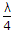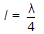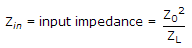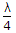# Electronics and Communication Engineering - Microwave Communication

### Exercise :: Microwave Communication - Section 2

36.

To couple a coaxial line to a parallel wire line it is best to use

 A. slotted line B. balun C. directional coupler D. λ/4 transformer

Answer: Option B

Explanation:

A balun gives 4 : 1 impedance transformation.

37.

Assertion (A): A quarter wave transformer is used to match a resistive load to a transmission line.

Reason (R): A quarter wave transformer is a transmission line of quarter wave length.

 A. Both A and R are correct and R is correct explanation of A B. Both A and R are correct but R is not correct explanation of A C. A is correct but R is wrong D. A is wrong but R is correct

Answer: Option B

Explanation:

A line of lengthis called quarter wavelength line. Such a line is used for impedance matching.

IfA quarter wave line can match a source impedance Zin with load impedance ZL by selecting a proper value of Z0 so as to satisfy equation.

Such a line is also calledtransformer.

38.

Assertion (A): Magnetron is generally used in n mode.

Reason (R): Frequency for p mode can be easily separated from adjacent modes.

 A. Both A and R are correct and R is correct explanation of A B. Both A and R are correct but R is not correct explanation of A C. A is correct but R is wrong D. A is wrong but R is correct

Answer: Option A

Explanation:

It is somewhat similar to TWT and can deliver microwave power over a wide frequency band.

It has an electron gun and a helix structure. However the interaction between electron beam and RF wave is different than in TWT.

The growing RF wave travels in opposite direction to the electron beam.

The frequency of wave can be changed by changing the voltage which controls the beam velocity.

Moreover the amplitude of oscillations can be decreased continuously to zero by changing the beam current.

It features are:

1. Frequency range - 1 GHz to 1000 GHz.

2. Power output - 10 mV to 150 mW (continuous wave) 250kW (pulsed).

It is used as signal source in transmitters and instruments.

39.

A balun should have

 A. low SWR at both ports B. high SWR at both ports C. high SWR at input port and low SWR at output port D. low SWR at input port and high SWR at output

Answer: Option A

Explanation:

Turn ratio is so selected as to give low SWR at both ports.

40.

Assertion (A): The velocity factor of a line is the ratio of wave velocity on the line to speed of light.

Reason (R): If the conductors of a line are immersed in a non magnetic insulating liquid, the wave velocity increases.

 A. Both A and R are correct and R is correct explanation of A B. Both A and R are correct but R is not correct explanation of A C. A is correct but R is wrong D. A is wrong but R is correct

Answer: Option C

Explanation:

R is wrong because wave velocity decreases.

#### Current Affairs 2021

Interview Questions and Answers